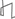Research Abstracts Home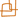CSAIL Digital Archive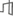Research Activities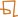CSAIL HomeResearch Abstracts - 2007IntroductionPerception & LearningPhysical, Biological & Social SystemsSystemsTheory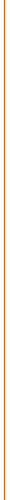### Bidimensionality Theory

#### Erik D. Demaine & MohammadTaghi Hajiaghayi

##### Overview

The theory of bidimensionality provides general techniques for designing efficient fixed-parameter algorithms and approximation algorithms for a broad range of NP-hard graph problems in a broad range of graphs. This theory applies to graph problems that are “bidimensional” in the sense that (1) the solution value for the k ×k grid graph and similar graphs grows with k, typically as Ω(k2), and (2) the solution value goes down when contracting edges and optionally when deleting edges in the graph. Many problems are bidimensional; a few classic examples are vertex cover, dominating set, and feedback vertex set.

##### Graph classes

Results about bidimensional problems have been developed for increasingly general families of graphs, all generalizing planar graphs.

The first two classes of graphs relate to embeddings on surfaces. A graph is planar if it can be drawn in the plane (or the sphere) without crossings. A graph has (Euler) genus at most g if it can be drawn in a surface of Euler characteristic g. A class of graphs has bounded genus if every graph in the class has genus at most g for a fixed g.

The next three classes of graphs relate to excluding minors. Given an edge e = {v,w} in a graph G, the contraction of e in G is the result of identifying vertices v and w in G and removing all loops and duplicate edges. A graph H obtained by a sequence of such edge contractions starting from G is said to be a contraction of G. A graph H is a minor of G if H is a subgraph of some contraction of G. A graph class C is minor-closed if any minor of any graph in C is also a member of C. A minor-closed graph class C is H-minor-free if HC. More generally, we use the term “H-minor-free” to refer to any minor-closed graph class that excludes some fixed graph H. A single-crossing graph is a minor of a graph that can be drawn in the plane with at most one pair of edges crossing. A minor-closed graph class is single-crossing-minor-free if it excludes a fixed single-crossing graph. An apex graph is a graph in which the removal of some vertex leaves a planar graph. A graph class is apex-minor-free if it excludes some fixed apex graph.

##### Bidimensional parameters

First we define “parameters” as an alternative view on optimization problems. A parameter P is a function mapping graphs to nonnegative integers. The decision problem associated with P asks, for a given graph G and nonnegative integer k, whether P(G) ≤ k. Many optimization problems can be phrased as such a decision problem about a graph parameter P.

Now we can define bidimensionality. A parameter is g(r)-bidimensional (or just bidimensional) if it is at least g(r) in an r × r “grid-like graph” and if the parameter does not increase when taking either minors (g(r)-minor-bidimensional) or contractions (g(r)-contraction-bidimensional). The exact definition of “grid-like graph” depends on the class of graphs allowed and whether we are considering minor- or contraction-bidimensionality. For minor-bidimensionality and for any H-minor-free graph class, the notion of a “grid-like graph” is defined to be the r × r grid, i.e., the planar graph with r2 vertices arranged on a square grid and with edges connecting horizontally and vertically adjacent vertices. For contraction-bidimensionality, the notion of a “grid-like graph” is as follows:

1. For planar graphs and single-crossing-minor-free graphs, a “grid-like graph” is an r ×r grid partially triangulated by additional edges that preserve planarity.
2. For bounded-genus graphs, a “grid-like graph” is such a partially triangulated r ×r grid with up to genus(G) additional edges ("handles").
3. For apex-minor-free graphs, a “grid-like graph” is an r ×r grid augmented with additional edges such that each vertex is incident to O(1) edges to nonboundary vertices of the grid. (Here O(1) depends on the excluded apex graph.)

Contraction-bidimensionality is so far undefined for H-minor-free graphs (or general graphs).

Examples of bidimensional parameters include the number of vertices, the diameter, and the size of various structures such as feedback vertex set, vertex cover, minimum maximal matching, face cover, a series of vertex-removal parameters, dominating set, edge dominating set, R-dominating set, connected dominating set, connected edge dominating set, connected R-dominating set, unweighted TSP tour (a walk in the graph visiting all vertices), and chordal completion (fill-in). For example, feedback vertex set is Ω(r2)-minor-bidimensional (and thus also contraction-bidimensional) because (1) deleting or contracting an edge preserves existing feedback vertex sets, and (2) any vertex in the feedback vertex set destroys at most four squares in the r ×r grid, and there are (r−1)2 squares, so any feedback vertex set must have Ω(r2) vertices. See [DFHT05b,DFHT04] for arguments of either contraction- or minor-bidimensionality for the other parameters.

##### Results

Bidimensionality builds on the seminal Graph Minor Theory of Robertson and Seymour, by extending some mathematical results and building new algorithmic tools. The foundation for several results in bidimensionality are the following two combinatorial results. The first relates any bidimensional parameter to treewidth, while the second relates treewidth to grid minors.

Theorem 1 [DH05b,DFHT04] If the parameter P is g(r)-bidimensional, then for every graph G in the family associated with the parameter P, tw\nolimits(G) = O(g−1(P(G))). In particular, if g(r) = Θ(r2), then the bound becomes tw\nolimits(G) = O(√P(G)).

Theorem 2 [DH05b] For any fixed graph H, every H-minor-free graph of treewidth w has an Ω(w) ×Ω(w) grid as a minor.

The two major algorithmic results in bidimensionality are general subexponential fixed-parameter algorithm, and general polynomial-time approximation scheme (PTASs):

Theorem 3 [DH05b,DFHT04] Consider a g(r)-bidimensional parameter P that can be computed on a graph G in h(w)  nO(1) time given a tree decomposition of G of width at most w. Then there is an algorithm computing P on any graph G in P's corresponding graph class, with running time [h(O(g−1(k))) + 2O(g−1(k))]  nO(1). In particular, if g(r) = Θ(r2) and h(w) = 2o(w2), then this running time is subexponential in k.

Theorem 4 [DH05a] Consider a bidimensional problem satisfying the “separation property” defined in [DH05a,DH]. Suppose that the problem can be solved on a graph G with n vertices in f(n,tw\nolimits(G)) time. Suppose also that the problem can be approximated within a factor of α in g(n) time. For contraction-bidimensional problems, suppose further that both of these algorithms also apply to the “generalized form” of the problem defined in [DH05a,DH]. Then there is a (1+ε)-approximation algorithm whose running time is O(n  f(n,O2/ε)) + n3 g(n)) for the corresponding graph class of the bidimensional problem.

The theorems above have many combinatorial and algorithmic applications. If we apply the parameter-treewidth bound of Theorem 1 to the parameter of the number of vertices in the graph, then we prove that every H-minor-free graph on n vertices has treewidth O(√n), thus (re)proving the separator theorem for H-minor-free graphs. If we apply the parameter-treewidth bound of Theorem 1 to the parameter of the diameter of the graph, then we prove a stronger form of Eppstein's diameter-treewidth relation for apex-minor-free graphs. (Further work shows how to further strengthen the diameter-treewidth relation to linear [DH04b].) The treewidth-grid relation of Theorem 2 can be used to bound the gap between half-integral multicommodity flow and fractional multicommodity flow in H-minor-free graphs. It also yields an O(1)-approximation for treewidth in H-minor-free graphs. The subexponential fixed-parameter algorithms of Theorem 3 subsume or strengthen all previous such results. These results can also be generalized to obtain fixed-parameter algorithms in arbitrary graphs. The PTASs of Theorem DH05a in particular establish the first PTASs for connected dominating set and feedback vertex set even for planar graphs. For details of all of these results, see [DH].

##### Future Directions

Several combinatorial and algorithmic open problems remain in the theory of bidimensionality and related concepts.

Can the grid-minor theorem for H-minor-free graphs, Theorem 2, be generalized to arbitrary graphs with a polynomial relation between treewidth and the largest grid minor? (The best relation so far is exponential.) Such polynomial generalizations have been obtained for the cases of “map graphs” and “power graphs” [DHK06]. Good grid-treewidth bounds have applications to minor-bidimensional problems.

Can the algorithmic results (Theorem 3 and Theorem DH05a) be generalized to solve contraction-bidimensional problems beyond apex-minor-free graphs? It is known that the basis for these results, Theorem 1, does not generalize [DFHT04]. Nonetheless, Theorem 3 has been generalized for one specific contraction-bidimensional problem, dominating set [DFHT05b].

Can we generalize the polynomial-time approximation schemes of Theorem DH05a to more general algorithmic problems that do not correspond directly to bidimensional parameters? One general family of such problems arises when adding weights to vertices and/or edges, and the goal is e.g. to find the minimum-weight dominating set. Another family of such problems arises when placing constraints (e.g., on coverage or domination) only on subsets of vertices and/or edges. Examples of such problems include Steiner tree and subset feedback vertex set.

For additional open problems and details about the problems above, see [DH].

##### References
[DFHT04]
Erik D. Demaine, Fedor V. Fomin, MohammadTaghi Hajiaghayi, and Dimitrios M. Thilikos. Bidimensional parameters and local treewidth. SIAM Journal on Discrete Mathematics, 18(3):501-511, December 2004.
[DFHT05a]
Erik D. Demaine, Fedor V. Fomin, MohammadTaghi Hajiaghayi, and Dimitrios M. Thilikos. Fixed-parameter algorithms for (k,r)-center in planar graphs and map graphs. ACM Transactions on Algorithms, 1(1):33-47, 2005.
[DFHT05b]
Erik D. Demaine, Fedor V. Fomin, MohammadTaghi Hajiaghayi, and Dimitrios M. Thilikos. Subexponential parameterized algorithms on graphs of bounded genus and H-minor-free graphs. Journal of the ACM, 52(6):866-893, 2005.
[DH]
Erik D. Demaine and MohammadTaghi Hajiaghayi. The bidimensionality theory and its algorithmic applications. Computer Journal. To appear.
[DH04a]
Erik D. Demaine and MohammadTaghi Hajiaghayi. Diameter and treewidth in minor-closed graph families, revisited. Algorithmica, 40(3):211-215, August 2004.
[DH04b]
Erik D. Demaine and MohammadTaghi Hajiaghayi. Equivalence of local treewidth and linear local treewidth and its algorithmic applications. In Proceedings of the 15th ACM-SIAM Symposium on Discrete Algorithms (SODA'04), pages 833-842, January 2004.
[DH05a]
Erik D. Demaine and MohammadTaghi Hajiaghayi. Bidimensionality: New connections between FPT algorithms and PTASs. In Proceedings of the 16th Annual ACM-SIAM Symposium on Discrete Algorithms (SODA 2005), pages 590-601, Vancouver, January 2005.
[DH05b]
Erik D. Demaine and MohammadTaghi Hajiaghayi. Graphs excluding a fixed minor have grids as large as treewidth, with combinatorial and algorithmic applications through bidimensionality. In Proceedings of the 16th Annual ACM-SIAM Symposium on Discrete Algorithms (SODA 2005), pages 682-689, Vancouver, January 2005.
[DHK06]
Erik D. Demaine, MohammadTaghi Hajiaghayi, and Ken-ichi Kawarabayashi. Algorithmic graph minor theory: Improved grid minor bounds and Wagner's contraction. In Proceedings of the 17th Annual International Symposium on Algorithms and Computation, volume 4288 of Lecture Notes in Computer Science, pages 3-15, Calcutta, India, December 2006.
[DHN+04]
Erik D. Demaine, MohammadTaghi Hajiaghayi, Naomi Nishimura, Prabhakar Ragde, and Dimitrios M. Thilikos. Approximation algorithms for classes of graphs excluding single-crossing graphs as minors. Journal of Computer and System Sciences, 69(2):166-195, September 2004.
[DHT05]
Erik D. Demaine, MohammadTaghi Hajiaghayi, and Dimitrios M. Thilikos. Exponential speedup of fixed-parameter algorithms for classes of graphs excluding single-crossing graphs as minors. Algorithmica, 41(4):245-267, February 2005.
[DHT06]
Erik D. Demaine, MohammadTaghi Hajiaghayi, and Dimitrios M. Thilikos. The bidimensional theory of bounded-genus graphs. SIAM Journal on Discrete Mathematics, 20(2):357-371, 2006.Computer Science and Artificial Intelligence Laboratory (CSAIL) The Stata Center, Building 32 - 32 Vassar Street - Cambridge, MA 02139 - USA tel:+1-617-253-0073 - publications@csail.mit.edu